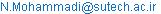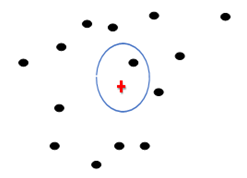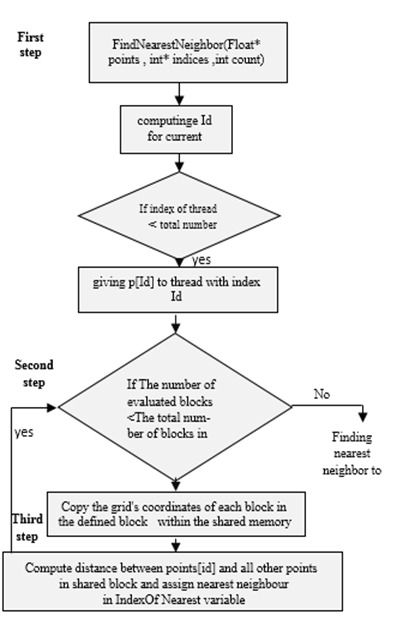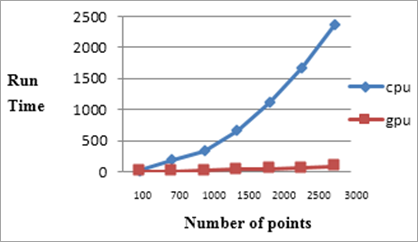American Journal of Software Engineering and Applications
Volume 5, Issue 3-1, May 2016, Pages: 10-14

Evaluation of GPU Performance Compared to CPU for Implementing Algorithms with High Time Complexity

Department of Computer Engineering, Shiraz University of Technology, Shiraz, IranNeda Mohammadi. Evaluation of GPU Performance Compared to CPU for Implementing Algorithms with High Time Complexity. American Journal of Software Engineering and Applications. Special Issue: Advances in Computer Science and Information Technology in Developing Countries. Vol. 5, No. 3-1, 2016, pp. 10-14. doi: 10.11648/j.ajsea.s.2016050301.13

Received: February 2, 2016; Accepted: February 6, 2016; Published: June 24, 2016

Abstract: Nowadays a number of applications with high volume of calculations are constantly increasing. Central Processing Unit (CPU) consists of finite number of cores. Degree of parallelism and implementation speed are issues that data high volume on CPU is low. Using the thread concept in programming, algorithms which have the parallelism capabilities, can be executed in parallel. There are many issues which in order to solving them, finding similar items in a metric space and grouping them in these issues is necessary. Computational complexity finding nearest neighbors is a challenge for run time. To evaluate the performance of GPUs speed in searching nearest neighbors, GPGPU and CUDA are used and compared with CPU usage. In this paper parallel implementation of the algorithm on GPU with access to its shared memory, is compared with parallel implementation of the algorithm on CPU through threads. It is understood that threads use graphics card's shared memory for communications, storing temporary data and retrieving data. Therefore, the parallelism on GPU is more useful than parallelism on CPU in High-Dimensional spaces. Finally, it is discussed that GPU reduces complexity to a considerable amount and is scalable.

Keywords: Nearest Neighbor, CUDA, GPU, Shared Memory, Parallelism

1. Introduction

Nowadays, there are many algorithms with high computational and time complexity. Also, there are many issues in computer science, which in order to be solved, in these issues, finding similar items in a metric space and grouping them is necessary. When dataset is small and its dimension is low, finding similar items is easy. But in many issues, such as image processing, data mining, machine learning etc. [1-2]. Finding similar items is required. In these issues, usually dimension and data volume is high. In this case, finding the nearest neighbors to each item has high time complexity. Therefore, the main and important issue, for searching nearest neighbor is presentation of a solution to decrease time complexity, when data dimension and volume is high. Searching the nearest neighbor algorithm, finds nearest neighbor to an item in a metric space. Parallel processing methods are an efficient solution to decrease complexity in implementation of nearest neighbor search. There are hundreds of cores in the architecture of the graphics processing unit, any core alone is able to carry out simple tasks. A series of multi-core constitute a multi-processor. Each multi-processor has an exclusive memory, such as shared memory, local memory and registers. Also any multi-processor has a controller and a dynamic ram. They are used as input and output functions, which run on the GPU . NVIDIA has provided the conditions that can run algorithms with high complexity by using your system's graphics processing units. These algorithms have the ability of parallelism. For this purpose, NVIDIA introduced CUDA technology [3-4-5]. Using CUDA can be written programs with both C and C++ language and run in parallel on the graphics processing unit. Therefore, GPU provides a scalable solution for nearest neighbor algorithm when data volume is high .

Another way for parallel implementation of the nearest neighbor search algorithm is utilization of thread in java programing. In this way, it can run algorithm in parallel on cores of central processing unit.

The number of cores in CPU is much fewer than GPU. GPU is composed of hundreds of small cores that are able to perform simple calculations. In this paper, the aim is evaluation of GPU performance compared to CPU, in order to implement algorithms with high complexity. For this purpose, two parallel implementation of nearest neighbor search (NNS) algorithm is performed [6-7]. In the first implementation, to parallel execution of this algorithm on GPU, CUDA technology is used. In the second implementation, the concept of threads in the java is used to parallelize the algorithms on the central processing unit (CPU). It is expected, implementation of NNS algorithm on GPU increases the speed of running and it is more efficient than parallel execution on the CPU.

The remainder of the article is structured as follow. In section 2, the related previous works is considered. In section 3 the nearest neighbor search algorithm is described. Proposed method for parallel implementation of NNS algorithm with accessing shared memory on GPU is presented and also, parallel implementation of this algorithm on CPU is expressed in section 4. The implementation results and comparison of two methods of implementing parallel algorithm is discussed in section 5. Finally, Section 6 draws the conclusion.

2. Related Studies

There have been several research studies in this field. In 2008, a method was introduced to improve efficiency in the running time for the serial algorithm on CPU. A parallel algorithm is to find k nearest neighbor to each item. This algorithm by using CUDA technology that creates trees with k degree. This method has low scalability .

In another study , NNS (Nearest Neighbor Search) algorithm is implemented on GPU, when GPU's shared memory is utilized; Parallelization of the algorithm on GPU using CUDA increases execution speed compared to serial implementation and also it is implemented on GPU without accessing shared memory.

In  'brute force' method is introduced. Author has reported, this method for implementing KNNS algorithm is more efficient than serial implementation. Also it is suggested that this method is faster than kd trees (trees with k degree).

Furthermore others  use a random algorithm called LSH (Locality Sensitive Hashing) for this purpose. This algorithm can find the nearest neighbors to a special point. This method decreases complexity. However with a low probability, it is not accurate in finding neighbors and does not guarantee the correct answer.

In this paper, serial KNN algorithm in  is used as a starting point. In the following , complexity of parallel implementation NNS algorithm on GPU and CPU is computed, then for both method, performance is measured.

3. Nearest Neighbor Search Algorithm

To implement nearest neighbor search algorithm, a set of points (p) in 3-dimensional space is introduced. p= {p1, p2,...,pn}. Here, the purpose is to find nearest neighbor for any point, such as 'q' in set of 'p' points. Different formula exists to calculate distance between points in metric spaces. In this paper, to compute distance between points, Euclidean distance formula is used. Figure1 shows k nearest neighbor issue where k is equal to 1.Figure 1. Black points are all points in set. Plus sign shows a point which should be found nearest point to it. Circle finds nearest neighbor to querying point.

Code 1. shows serial pseudo code for nearest neighbor search algorithm.

//curPoint is queryPoint

For(int curPoint=0;curPoint<count;curPoint++)

For(int i=0;i<count;i++)

Compute all distance between query curpoint and rj  , j/span>[1,count]

Code 1. serial pseudo code for nearest neighbor search algorithm

In this pseudo code, curpoint is a query point that must be found nearest neighbor to it. The total number of points set is assigned in 'count' variable. High complexity is a key issue in the nearest neighbor search algorithm. Due to the pseudo-code in Code 1, finding nearest neighbor for a special point is order of O(n). So, finding the nearest neighbor for any point of this set of points is of degree O(n^2). When the total number of points in the set is high, complexity for this algorithm also is high. Equation 1 shows, how the distance between two points in three-dimentional space is calculated.

Distance=Sqrt((x1-x2)2+(y1-y2)2+(z1-z2)2)          (1)

All implementation is done on a system with the properties provided in Table 1.

Table 1. System properties.

 RAM OS Graphics card CPU 3GB Windows 7 GeForce 9300 MG Intel 2.40 GHZ

3.1. Parallelism Nearest Neighbor Search, Using Threads in Java Programing

The purpose of parallelizing an algorithm is for the better use of the system resources and increasing the speed and efficiency. In this type of programming, part of the program that have parallelism capability, have been divided into several sub-programs. Then, they are running on multi-core processor by multi-threading. The most important reason to use parallel programming is, speed increasing in execution of the program. Threads, during execution of program, make overlap between execution of processors and input/output operations. Unlike CPU, GPU has a parallel architecture. It uses multiple threads simultaneously, thus general problem on graphic cards (called GPGPU)  is solved.

3.2. Parallelism Using CUDA Technology on GPU

GPU is used to solve issues with high complexity. This is a main difference between utilization of GPU and CPU. In GPU compared to CPU, more transistors assigned for calculations. Using CUDA technology, NVIDIA's graphics cards are used to execute the computational algorithms. The Number of cores in GPU is much more than CPU. It causes the speed of GPU be higher than CPU .

3.3. Parallelism of the Nearest Neighbor Search Algorithm

The purpose is to increase the runtime speed runtime in execution of nearest neighbor search algorithm. For this purpose, two methods are used. In the first method, algorithm runs on GPU with access to GPU's shared memory and use of CUDA technology. The second method, execution of the algorithm in parallel on CPU's cores using thread in java programming. In both methods, for any point in a three-dimensional space, response time is measured. Finally, the performance for both methods is evaluated.

In this paper, definition of response time is elapsed time between start and end finding operation. To reach to this purpose, many factors exist such as processor type, number of processor, type of graphic card and most important is presentation of a method for parallelism . Considering pseudo code in Code 1, to find nearest neighbor to any point, there are two loops. So complexity of algorithm is degree of O(n^2). Due to the pseudo code, it can be understand, which NNS algorithm has the good capability for parallelism. Parallelism of NNS algorithm should be done, in a manner that overhead associated to thread connections with global memory as much as possible be reduced and dose not overcome on runtime. This is not possible to parallelize the inner loop. Because calculation to find nearest neighbor is depending on calculation of distance for other points. There are many ways to break issue and give it to threads. Here, the issue is broken and given to threads. Number of threads is equal to number of points in set of points. Any thread is assigned to any point. Therefore any thread finds nearest point to itself.

4. Parallelism

4.1. Parallelism Using GPU's Shared Memory

One of the possibilities of GPU is shared memory for each block. Size of this memory is small, but it has high speed. Shared memory is used to communicate between threads in a block or save and retrieve data that frequently has been accessed by the threads.

If shared memory does not exist, threads to communicate with each other or to save and retrieve data, must be access to GPU's global memory. Speed of GPU's global memory is very low. Therefore, it greatly reduces the speed of execution. To do phases in Figure 2, at first it must be defined "shared-point" array (including threads in a block). Every time, kernel transmits a block from global memory to shared memory, each thread in block, is corresponding to a point from set of points. So it finds nearest neighbor to itself between all threads in shared memory's block.Figure 2. Implementation of parallelism algorithms using shared memory.Figure 3. Run time in implementation of both method in parallel.

1.   Calculates its distance with all points within the shared memory

2.   Finds nearest point to itself

3.   Keeps its nearest neighbor distance in 'IndexOfNearest' variable. The number of read operations from grid and copy at shared memory is equal to the number of blocks at the grid.

Finally, every thread will find nearest point to itself. In this implementation, each block within the grid is consisting of 320 threads , which are determined by programmer. To implement operations in Fig 3 just a loop is needed and complexity is O(n).

At CUDA, like other parallelism technologies, when threads are used, they use the shared memory, thus competitive conditions will be created which leads to the hazard. To avoid hazard, syncthreads() function is used instead semaphores [14-15]. This function is as a barrier and no thread at a block will not pass of barrier, until, all threads into block reach to this point.

4.2. Parallelism Using Threads in Java on CPU

In this implementation, NNS algorithm in parallel is performed on CPU. pseudo code is presented in Code 2.

In main method, the numbers in the 'p' array is generated by Random function in range [-5000, +5000]. 'array' method creates an array of threads by Thread class in java. Number of threads is equal to the number of points at set. That part of the code which can be parallelized, should be implemented by 'run' method.

5. The Implementation Results

To evaluate the efficacy of parallelism, the number of points in set 100,700,1000,1500,2000,2500,3000 is considered, because these numbers often are used in real applications. For each of these sets, the program is run 20 times. As previously mentioned, the numbers into array are generated randomly in the range [-5000, +5000].

Finally, for each of this set of points which has been implemented 20 times, an arithmetic mean has been considered as a run time factor. Figure 3 shows, the execution time for different number of points in implemented of two algorithm.

Public class FindClosest

{

Public void run(){

//Parallel Compute nearest distance for any thread}}

Public void array{

//create a object array of thread

//number of threads is equal number of points

//assign any thread to a point for Compute nearest neighbor own

Public static void main(String [] args){

//initial array of points with random number in range [-5000,+5000]

//Show Elapsed time

//Show nearest neighbor for any points}}

Code 2. pseudo code for parallelism of algorithm on cpu using thread in java programming

As can be seen in Figure 3, implementation on GPU, for data high volume has very high performance compared to implementation of same algorithms on CPU.

6. Discussion and Conclusion

According to the results that were achieved, it can be clearly seen that the parallel algorithm on GPU especially when data volume is high has a significant impact in reducing execution time. As previously mentioned, when data volume is small, solving the nearest neighbor search issue is easy. However, when the data volume is large, time complexity of algorithm is high. Therefore the suggested method has the best implementation in terms of execution cost, efficient use of hardware and scalability and unlike the mentioned method in , returns a definitive answer as the nearest neighbor.

References

1. M., Guevara, G., Chris, K., Hazelwood, and K., Skadron. "Enabling task parallelism in the cuda scheduler." In Workshop on Programming Models for Emerging Architectures, 2009, pp. 69-76.
2. V., Garcia,, E., Debreuve, F., Nielsen, and M., Barlaud,. "K-nearest neighbor search: Fast GPU-based implementations and application to high-dimensional feature matching". In Image Processing (ICIP), 2010, September. 17th IEEE International Conference on (pp. 3757-3760).
3. CUDA C Programming Guide: CUDA Toolkit Documentation,http://docs.nvidia.com/cuda/,cuda-c-programming-guide/index.html.
4. S., Liang, Y., Liu, C. Wang, and L., Jian,. "Design and evaluation of a parallel k-nearest neighbor algorithm on CUDA-enabled GPU". In Web Society (SWS), 2010 IEEE 2nd Symposium on (pp. 53-60). IEEE, 2010, August.
5. Hawick, Kenneth A., Arno Leist, and Daniel P. Playne."Parallel graph component labelling with GPUs and CUDA". Parallel Computing (2010) 36, no. 12 655-678.
6. S., Liang, Y., Liu, C., Wang, and L., Jian, 2009, October. "A CUDA-based parallel implementation of K-nearest neighbor algorithm". In Cyber-Enabled Distributed Computing and Knowledge Discovery, 2009. CyberC'09. International Conference on (pp. 291-296). IEEE
7. D., Qiu, S., May, & A., Nüchter. "GPU-accelerated nearest neighbor search for 3D registration". In Computer Vision Systems, 2009. (pp. 194-203). Springer Berlin Heidelberg.
8. V., Garcia, E., Debreuve, & M.Barlaud, "Fast k nearest neighbor search using GPU". In Computer Vision and Pattern Recognition Workshops, 2008. CVPRW'08. 2008, June. IEEE Computer Society Conference on (pp. 1-6). IEEE.
9. N. Mohammadi. "Presentation of a Parallel Algorithm for Nearest Neighbor Search on GPU Using CUDA". Current Trends in Technology and Sciences (CTTS)2015.
10. J., Nickolls, I., Buck, M., Garland, & K.Skadron, "Scalable parallel programming with CUDA". Queue, 6(2), 2008. 40-53.
11. Nourzad."The introduction of context-sensitive hashing algorithm for finding nearest neighbors in high-dimensional spaces". Amirkabir University of Technology, (2010).
12. X., Wu, V., Kumar, J. R.,Quinlan, J., Ghosh, Q., Yang, H., Motoda, & D. Steinberg."Top 10 algorithms in data mining. Knowledge and Information Systems", 2008.14(1), 1-37.
13. S., Liang, C., Wang, Y., Liu, and L., Jian,. "CUKNN: A parallel implementation of K-nearest neighbor on CUDA-enabled GPU". In Information, Computing and Telecommunication, 2009. YC-ICT'09. 2009, September. IEEE Youth Conference on (pp. 415-418).
14. A.Neumann, " Parallel reduction of multidimensional arrays for supporting online analytical processing (olap) on a graphics processing unit (gpu)".The University of Western Australia,2008.
15. S. Ryoo, C. I Rodrigues, S. S Baghsorkhi,S. S Stone, D. B.Kirk,, & W. M. W. Hwu, "Optimization principles and application performance evaluation of a multithreaded GPU using CUDA"..(2008, February) In Proceedings of the 13th ACM SIGPLAN Symposium on Principles and practice of parallel programming. (pp. 73-82). ACM.

 Contents 1. 2. 3. 3.1. 3.2. 3.3. 4. 4.1. 4.2. 5. 6.
Article ToolsAbstractPDF(1029K)